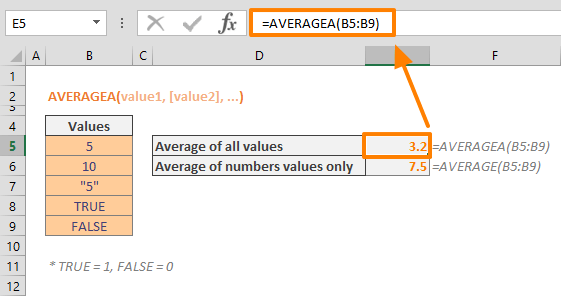The AVERAGEA in Excel is a Statistical function that can calculate and return the average (arithmetic mean) of the values of a range of cells. AVERAGEA differs from the AVERAGE function in its ability to include numbers in string format and logical values in the calculation. In this guide, we’re going to show you how to use the AVERAGEA in Excel, and also go over some tips and error handling methods.

## Supported versions

• All Excel versions

## AVERAGEA in Excel Syntax

AVERAGE(value1, [value2], …)

## Arguments

 value1 The first value or values you want to calculate. You can supply a static value like 4, a cell reference like B6, or a cell range like B2:B8. [value2], … Optional. The second and subsequent value or values you want to calculate, up to 255 values.

## Examples for AVERAGEA in Excel

You can use the AVERAGEA function by supplying values as arguments. Although you can use any static value, the common practice is using a range of cells. The following example demonstrates a basic use case for AVERAGEA in Excel, and how it differs from the AVERAGE function.

=AVERAGEA(B5:B9)While AVERAGEA returns 3.2, the AVERAGE function returns 7.5. The reason for the difference in the outcomes is because of the values after 5 and 10. AVERAGEA evaluates "5", TRUE and FALSE as 5, 1 and 0.

## Tips

• We recommend using AVERAGEA in Excel over the AVERAGE function for when you want to evaluate the logical values TRUE and FALSE, as well as numbers that are represented as text.
• Logical values are evaluated as 1 and 0 which stand for TRUE and FALSE
• If you want to calculate the average of the values that meet certain criteria, use the AVERAGEIF function or the AVERAGEIFS function.